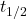## a compound decomposes by a first-order process. if 17.0% of the compound decomposes in 60.0 minutes, the half-life of the compound is

Question

a compound decomposes by a first-order process. if 17.0% of the compound decomposes in 60.0 minutes, the half-life of the compound is

in progress 0
6 months 2021-07-31T03:31:27+00:00 1 Answers 21 views 0

## Answers ( )

the half-life of the compound is 223 minutes

Explanation:

Given the data in the question;

compound decomposes by a first-order process,

17% of compound decomposes in 60 minutes,

the half-life of the compound = ?

Since its a first order reaction, we use the expression;

k = 1/t ln( Co/Cf )

given that; t = 30 minutes, Cf = 1 – 17% of Co = 1 – 0.17 of Co = 0.83Co

we substitute

k = ( 1 / 60 min ) ln( Co / 0.83Co )

k =  ( 1 / 60 min ) ln( 1 / 0.83 )

k = 0.016667 × 0.186329578

k = 0.003105555

so get the half life;= 0.693 / k

we substitute= 0.693 / 0.003105555= 223.14 ≈ 223 minutes

Therefore, the half-life of the compound is 223 minutes